SSC CGL Posts Preparation: Statistics - Study24x7New to Study24X7 ?

# SSC CGL Posts Preparation: Statistics

Updated on 15 February 2020Harshit Verma
Updated on 15 February 2020## SSC CGL Posts Preparation: Statistics

SSC CGL 2020 preparation for statistics includes conceptual knowledge of the subject. It is not mandatory subject for all candidates. Mock tests in English and Hindi for competitive exams will do a great help to clear the concepts and practice regularly.

SSC  Preparation: Moments, Skewness and Kurtosis

Moments: It is characteristic of distribution.

·      This statistical term is used to define moments of forces in physics.

·      These are the constant value which gives idea about type of distribution and its nature.

·      It is denoted by µ.

Skewness: It is lack of symmetry in distribution.

·      Here values of mean, median and mode are not same.

·      Skewness is either positive or negative

Positive Skewness: where value of mean is larger than mode. Here median lies between mean and mode.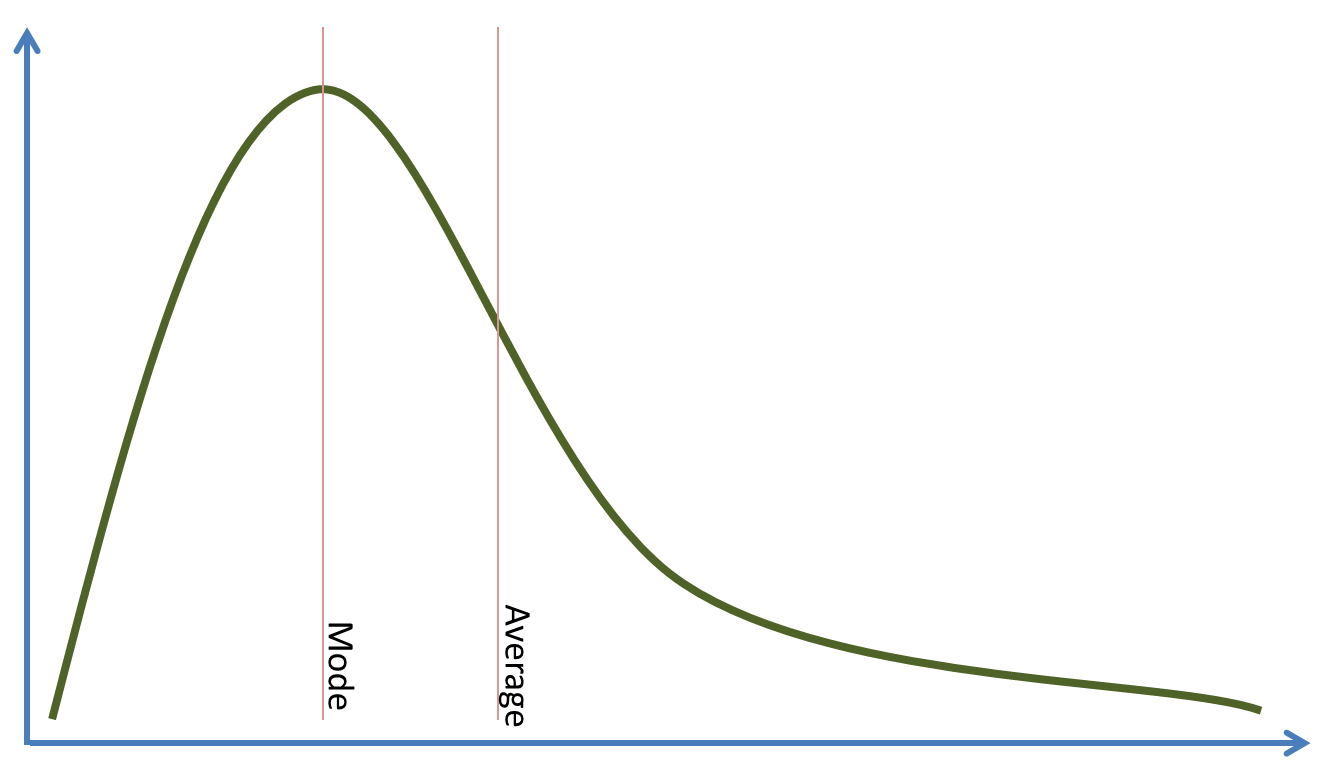Negative Skewness: Where value of mode is larger than mean. Here also median lies between mean and mode.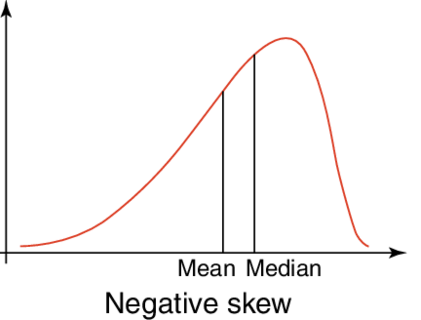Kurtosis: It describes in a frequency curve.

·      It shows the degree of concentration either peaked or flat region of a frequency curve.

·      This concept is not used to analyze business data generally.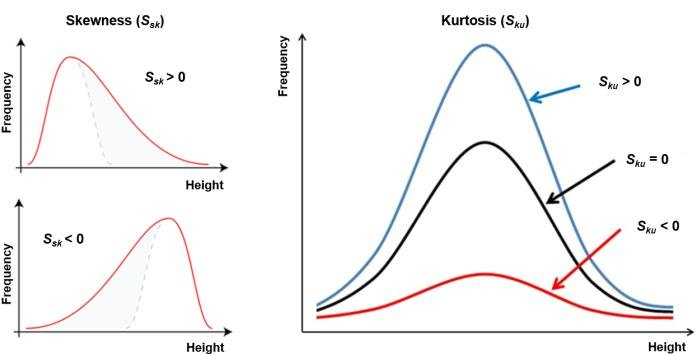SSC Preparation: Correlation and Regression

These two are used to analyze distribution of multiple variable or multivariate distributions. Both used to quantify the direction and strength of the relationship between two numerical values.

Correlation: It is a statistical term to determine the association between two variables.

·      It represents linear relation.

·      It gives a numerical value which shows relationship between two variables.

·      The degree of association is measured by a coefficient called correlation coefficient (r).

·      On the name of its originator it is also called Pearson's correlation coefficient.

Regression: It describes how an independent variable is numerically related to a dependent variable.

·      Here both variables are different.

·      It is used to find the value of random variables on the basis of a given fixed variable.

·      It produces an equation.

·      In simple linear regression, the model used to describe the relationship between a single dependent variable y and a single independent variable.

Example: y= a + bx; where x is an independent variable and y is a dependent variable.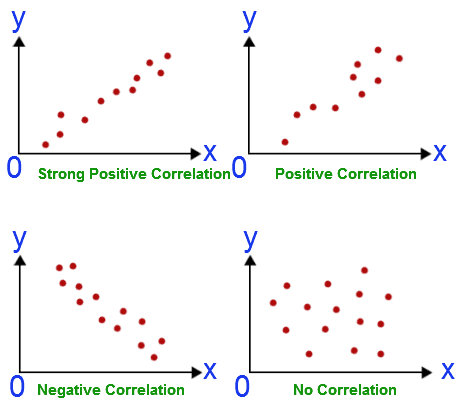SSC Preparation: Random Variables

·      It is a variable whose values are numerical outcomes of a random phenomenon.

·      A random variable is required to be measurable

·      Its mathematical application is in probability theory.

·      There are two types of random variables present called discrete and continuous.

Discrete Random Variable:  It takes finite or countable number of distinct values into account like students in a class. Example: 0, 1, 2….n.

·      It is defined at specific value.

·      It allows the computation of probabilities for individual integer values.

·      Possible values of the random variables are then treated as a sample space.

Continuous Random Variable: It takes indefinite number of values for measurements.

·      These are the variables whose cumulative distribution function is continuous everywhere.

·      It is defined over an interval.

·      It is represented by area under a curve which is mathematically known as integral.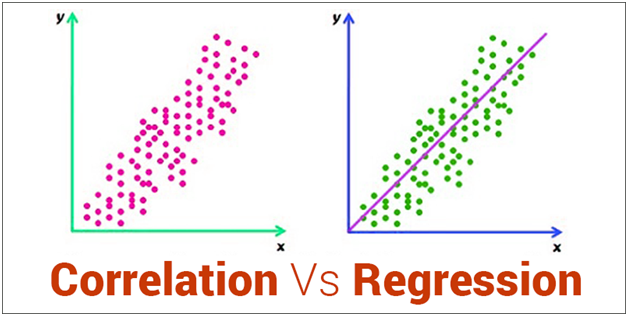These are few simple last minute revision roundups for SSC-CGl2020.

## All the best for SSC-CGL2020

Write a comment...Trending ArticlesBy CBSE CLASS 8By CBSE CLASS 10By CBSE CLASS 7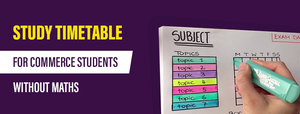By K-12-Commerce
Related Posts